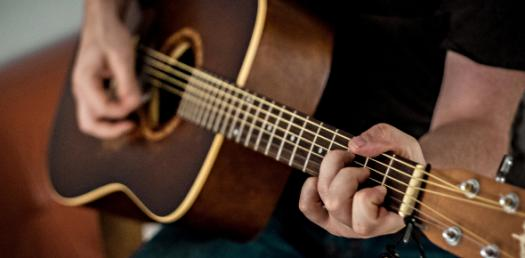# Take This Trivia Quiz On Guitar Chords!

12 Questions | Total Attempts: 1204SettingsCreate your own QuizTake this trivia quiz on guitar chords. If you have a dream of becoming a rock star, you will either be the voice, the drummer, or the guitar player. Without background sounds, the singer wouldn’t have the beat, so the quiz below is for those of you who are learning how to play the guitar. Take it up and have fun learning!

• 1.
What key are C and D chords in ?
• A.

C

• B.

D

• C.

G

• D.

F

• 2.
What key are C and D chords in ?
• A.

G

• B.

C

• C.

D minor

• D.

F

• 3.
What key is G chord in?
• A.

Key of G, C and D

• B.

Key of G

• C.

Key of G and D

• D.

Key of G and C

• 4.
What key are F and G chords in ?
• A.

F

• B.

G

• C.

F and G

• D.

C

• 5.
What are the minor chords in the key of G ?
• A.

Em,Bm and Am

• B.

Gm, Cm and Fm

• C.

Only Gm

• D.

There are none.

• 6.
What are the notes in a C chord?
• A.

C,D,E,F,G,A,B,C

• B.

C

• C.

C,D,E

• D.

C,E,G

• 7.
What key are Am and D in ?
• A.

A minor

• B.

D major

• C.

A minor and D major

• D.

G major

• 8.
What key are Em and A in?
• A.

D major

• B.

E minor

• C.

A major

• D.

E major

• 9.
What keys are G and C chord in ?
• A.

G major

• B.

G major and C major

• C.

G major, C major and F major

• D.

G major, E minor and C major

• 10.
What chords are in the key of F?
• A.

F,C and G

• B.

F,Dm,Bb,Gm,C,Am

• C.

F,Bb and C

• D.

F,Bb and Eb

• 11.
What is a key ?
• A.

A group of chords

• B.

A group of chords that are made from notes of a common scale.

• C.

A group of chords and the scale that the chords are made from.

• D.

The notes of a scale.

• 12.
How many keys are there?
• A.

There are 24, 12 minor and 12 major keys.

• B.

There are 12,one for each note name.

• C.

There are 7 natural keys and 5 sharp or flat keys.

• D.

There are 7, one for each note name A B C D E F G.Back to top
×

Wait!
Here's an interesting quiz for you.